# SOCR EduMaterials Activities BoxPlot

## Summary

This activity describes the construction of the box-and-whisker plot (or simply box plot) in SOCR. The applets can be accessed at SOCR Charts under the Miscellaneous folder.

## Goals

The aims of this activity are to:

• show the importance of the box plot in exploratory data analysis (EDA)
• illustrate how to use SOCR to construct a box plot
• present some unusual pathologies of a box plot

## Background & Motivation

The box plot (or box-and-whisker-plot), invented by John Tukey in 1977, is an efficient way for presenting data, especially for comparing multiple groups of data. In the box plot, we can mark-off the five-number summary of a data set (minimum, 25th percentile, median, 75th percentile, maximum). The box contains the $$50 %$$ of the data. The upper edge of the box represents the 75th percentile, while the lower edge is the 25th percentile. The median is represented by a line drawn in the middle of the box. If the median is not in the middle of the box then the data are skewed. The ends of the lines (called whiskers) represent the minimum and maximum values of the data set, unless there are outliers. Outliers are observations below $$Q_1 -1.5 (IQR)$$ or above $$Q_3 + 1.5(IQR)$$, where $$Q_1$$ is the 25th percentile, $$Q_3$$ is the 75th percentile, and $$IQR=Q_3-Q_1$$ (called the interquartile range). The advantage of a box plot is that it provides graphically the location and the spread of the data set, it provides an idea about the skewness of the data set, and can provide a comparison between variables by constructing a side-by-side box plots.

## Examples & Exercises

• Example 1: Go to the SOCR Charts and first, click on the Miscellaneous folder and then on BoxAndWhiskerChartDemo1. In the Demo1 box plot, we can see side-by-side box plots of two categories for each of three series. These demonstration data can be viewed by clicking on DATA. Clicking on MAPPING you can choose the variables. Clicking on SHOW ALL the applet will present the graph, the data, and the mapping environment. Let’s clear this data set (click on CLEAR) so that we can enter our own data. After you click on CLEAR button, click on the DATA tab to enter data into the spreadsheet. The following data will be entered (don’t forget to separate the data by commas!):
 C1 C2 C3 Series 1 1,2,3,4,5,6 2,4,6,8,10,12 Series 2 3,4,5,6,7,8 6,8,10,12,14,16,18 Series 3 5,6,7,8,9 10,16,18,20,22

When you finish entering your data, click on MAPPING to select the series and categories, and finally click on UPDATE_CHART to view the box plots. The following snapshot shows how the above data entered into SOCR: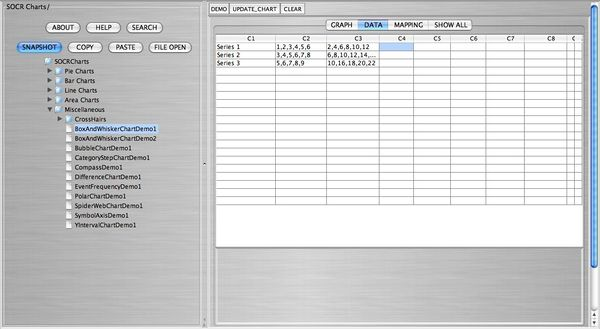The following snapshot shows the mapping of the data: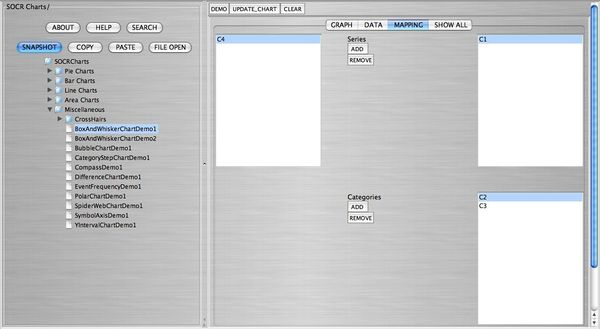The following snapshot shows the side-by-side box plots: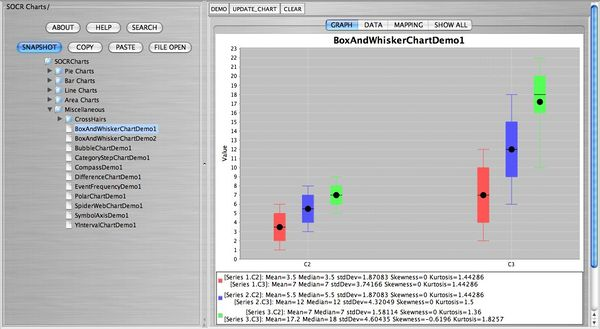The following snapshot shows the data, the mapping, and the box plots in one screen: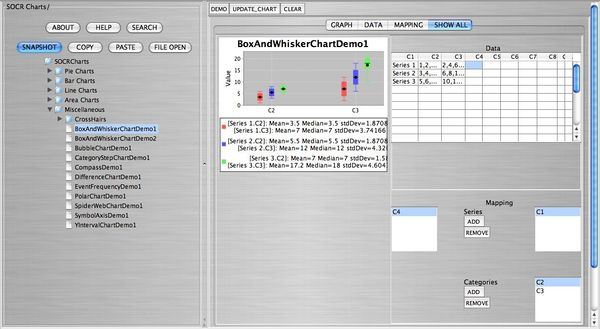• Example 2:

If we are working with a single variable, we can use the BoxAndWhiskerChartDemo2. Double click this link to see the demonstration of the construction of the box plot with one variable. As we did in example 1, we will enter our own data. Click on CLEAR to enter your data in the spreadsheet. The data we want to enter are the following: 60, 95, 72, 87, 88, 75, 76, 91, 100, 58, 78, 81, 73, 94, 65.

When you finish entering your data, click on MAPPING to select the category (here only C1), and finally click on UPDATE_CHART to view the box plot.

The following snapshot shows how the above data entered into SOCR: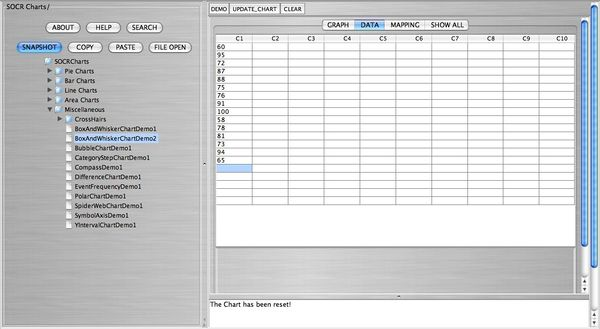The following snapshot shows the mapping of the data: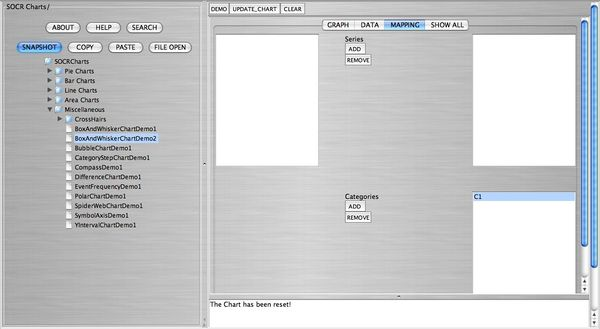The following snapshot shows the box plot: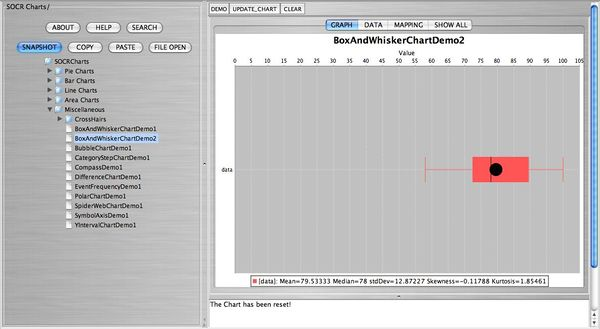The following snapshot shows the data, the mapping, and the box plots in one screen: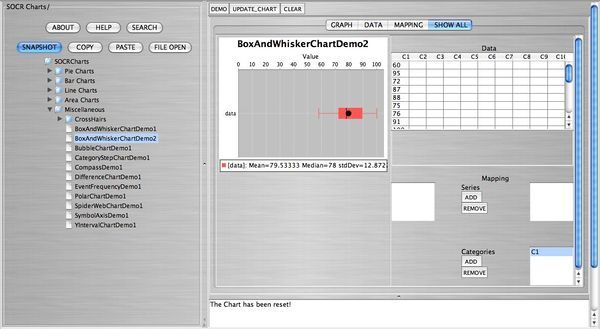### Box Plot Pathologies

Box plots can show unusual pathologies. For the following box plots, enter the data in the SOCR Charts spreadsheet that created them.

• Example 1: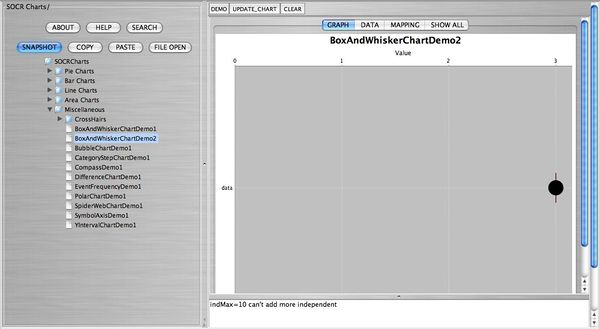• Example 2: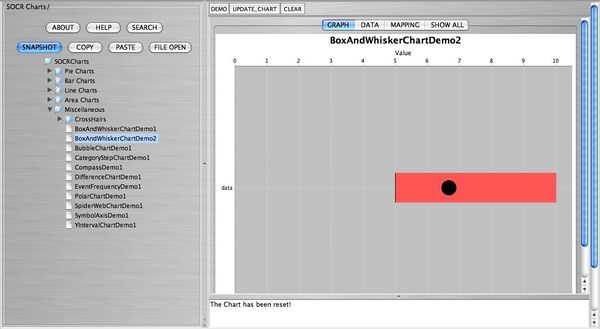• Example 3: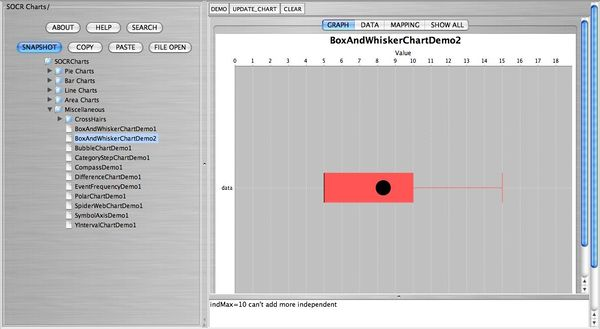• Example 4: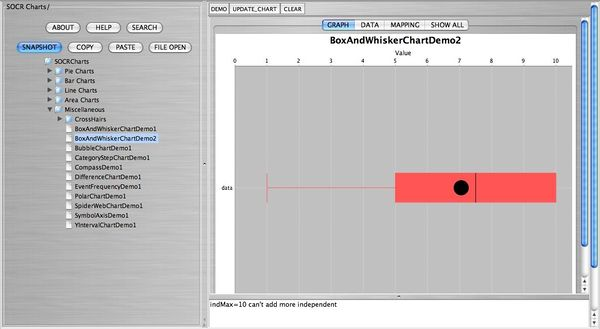• Example 5: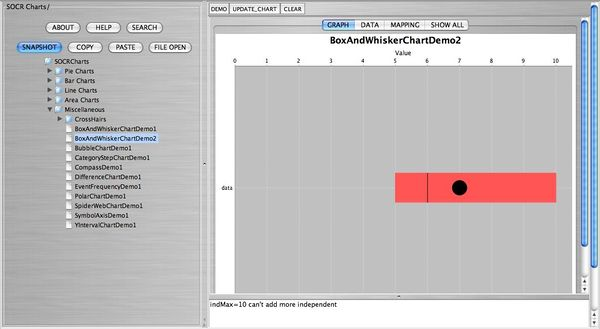## Other Forms of Data

Alternatively, the user can import data by clicking on FILE OPEN. Note here that the data must be saved previously as a comma delimited (CSV) in order to be accessed in SOCR.